### Home > CALC > Chapter 1 > Lesson 1.2.3 > Problem1-51

1-51.
1.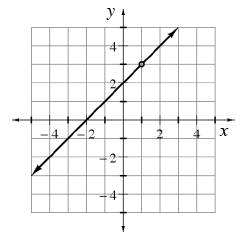Analyze the graph of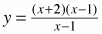at right. Homework Help ✎

1. What does y approach as x → ∞ ?

2. What does y approach as x → -∞ ?

3. What does y approach as x → l (from the left)?

4. What does y approach as x → 1+ (from the right)?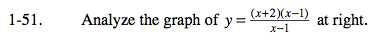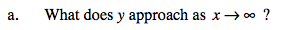The graph appears to be the linear function y = x + 2, with a hole at (1,3).

Just like the graph of y = x + 2, this function will go up (y →+∞) as we move to the right (x →∞)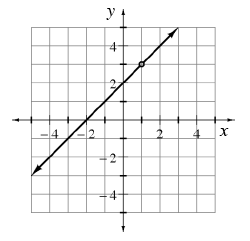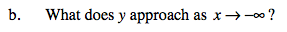Refer to the hint in part (a).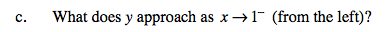As the graph approaches the hole from the left (x → −1), what y-value does it approach?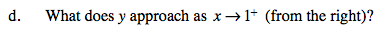Refer to the hint in part (c).